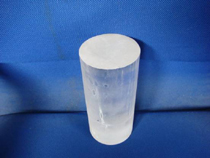# LBO Crystal

Lithium Tetraborate Crystal, Li2B4O7 (LBO) with its excellent piezoelectricity has been widely and extensively developed since the last two decades. This piezo crystal is ideal for the production of SAW filters, resonators, and other high frequency devices that feature low density, weak Temperature Coefficient of Delay (TCD), and SAW high coupling factor (k2), and unnecessary polarization before using.

Specifications:Crystal Orientation: <110>
Electric Constant: e33 = 0.91c/m
Coefficient of Dielectric Loss: εT33 = 10.1
Sound Propagation Velocity: 340m/s
Elastic Constant: CE33 = 5.42×1010N/m2, K33 = 0.42, K = 20.7%

 Point Group: 4mm Lattice Constant (A): a = 9.475 , c = 10.283 Density: 2.45g/cm3 Hardness (Mho) ~6 Melting Point (°C) 917 Dielectric Constant E11 = 3.5 E33 = 8.2 Dielectric Constant: e11/eo 51.7 e33/eo 44.5 Piezoelectric Strain Constant: D15 8.07 (x10-12C/M) D33 0.8 (x10-12C/M) Temperature Coefficient of Delay: 0 (x10-6 /°C @ 25°C) SAW Coupling Factor (k2): 0.7-1.6 SAW Velocity (m/s): 3402 – 3864 Coefficient of Thermal Expansion: a11 = 11.1×10-6/°C a33 = 3.7×10-6/°C Solubility (m/h): in Pure Water a = 0.04 c = 0.08 in Concentrated HNO3 60 in KOH 0.4 in C3HCl3 0